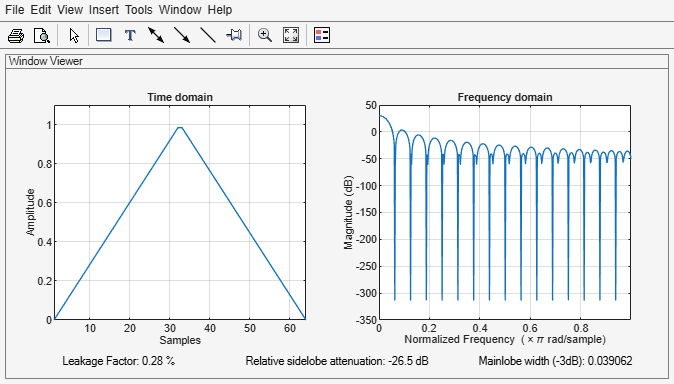# bartlett

Bartlett window

## Syntax

``w = bartlett(L)``

## Description

example

````w = bartlett(L)` returns an `L`-point symmetric Bartlett window.```

## Examples

collapse all

Create a 64-point Bartlett window. Display the result using `wvtool`.

```L = 64; bw = bartlett(L); wvtool(bw)```## Input Arguments

collapse all

Window length, specified as a positive integer.

Data Types: `single` | `double`

## Output Arguments

collapse all

Bartlett window, returned as a column vector.

## Algorithms

The following equation generates the coefficients of a Bartlett window:

`$w\left(n\right)=\left\{\begin{array}{ll}\frac{2n}{N},\hfill & 0\le n\le \frac{N}{2},\hfill \\ 2-\frac{2n}{N},\hfill & \frac{N}{2}\le n\le N.\hfill \end{array}$`

The window length $L=N+1$.

The Bartlett window is very similar to a triangular window as returned by the `triang` function. However, the Bartlett window always has zeros at the first and last samples, while the triangular window is nonzero at those points. For odd values of `L`, the center `L-2` points of``` bartlett(L)``` are equivalent to `triang(L-2)`.

Note

If you specify a one-point window (`L = 1`), the value `1` is returned.

 Oppenheim, Alan V., Ronald W. Schafer, and John R. Buck. Discrete-Time Signal Processing. Upper Saddle River, NJ: Prentice Hall, 1999, pp.